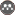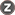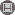Volume 4, Issue 2 (Summer 2017)                   johe 2017, 4(2): 41-52 | Back to browse issues page

BibTeX | RIS | EndNote | Medlars | ProCite | Reference Manager | RefWorks
Send citation to:Bakbaki A, Nabhani N, Anvaripour B, Shirali G. Probabilistic risk assessment using fuzzy fault tree analysis based on two types of failure possibility distributions in process industries. johe. 2017; 4 (2) :41-52
URL: http://johe.umsha.ac.ir/article-1-267-en.html
, anvaripour@put.ac.ir
Abstract:   (3444 Views)

Introduction: Probabilistic risk assessment using fault tree analysis is an effective tool to assess occurrence probability of hazardous events in chemical process industries. Unfortunately, the failure occurrence probability of the basic events are often not available in process industries. The aim of this study is how calculate basic events failure occurrence probability when basic events do not have precise failure data.
Methods: In this study, the proposed risk assessment framework was based on five-scale and six-scale fuzzy-based approaches. Firstly, the fault tree diagram was constructed using the risk identification methods. Then, by applying the two types of failure possibility distributions, failure occurrence probability of the basic events were calculated. Finally, using the importance measure analysis, critical minimal cut sets were ranked.
Results: Results show that the failure occurrence probabilities calculated by the five-scale and six-scale approaches for the 32 basic events were closed to each other. The occurrence probability of the top event calculated using the five-scale and six scale approaches were 3.64E-04 and 4.76E-04 at year, respectively. After ranking of the MCSs based on their calculated importance measures, the process failures have been determined as the critical causes of the TE.
Conclusions: The results show that the fuzzy-based approach seems to be a good alternative for conventional approach of the FTA to deal with basic events which do not have failure rate data to obtain their failure occurrence probabilities. This study has validated the consistency of fuzzy-based approach, to assess basic events failure occurrence probabilities.